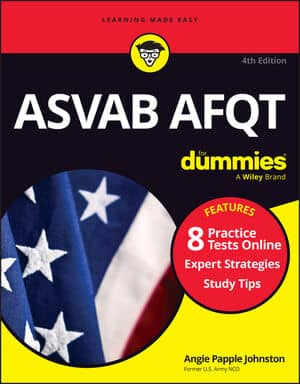##### ASVAB AFQT For DummiesA line score combines various standard ASVAB scores to see which jobs or training programs you qualify for. The standard scores are your scores on the individual ASVAB subtests (with Word Knowledge and Paragraph Comprehension combined as a Verbal Expression score):

• General Science (GS)

• Arithmetic Reasoning (AR)

• Auto & Shop Information (AS)

• Mathematics Knowledge (MK)

• Mechanical Comprehension (MC)

• Electronics Information (EI)

• Assembling Objects (AO)

• Verbal Expression (VE), the sum of Word Knowledge (WK) and Paragraph Comprehension (PC)

Each of the military services computes its line scores differently. Some calculations even include dummy scores — average scores received by thousands of test takers — for Numerical Operations (NO) and Coding Speed (CS), subtests that are no longer part of the ASVAB.

## Line scores and the Army

To compute line scores for job qualification, the Army combines the various scores into ten separate areas by simple addition of the ASVAB standard scores.

Line Score Standard Scores Used Formula Used
Clerical (CL) Verbal Expression (VE), Arithmetic Reasoning (AR), and Mathematics Knowledge(MK) VE + AR + MK
Combat (CO) Arithmetic Reasoning (AR), Coding Speed (CS), Auto & Shop Information (AS), and Mechanical Comprehension (MC) AR + CS + AS + MC
Electronics (EL) General Science (GS), Arithmetic Reasoning (AR), Mathematics Knowledge (MK), and Electronics Information (EI) GS + AR + MK + EI
Field Artillery (FA) Arithmetic Reasoning (AR), Coding Speed (CS), Mathematics Knowledge (MK), and Mechanical Comprehension (MC) AR + CS + MK + MC
General Maintenance (GM) General Science (GS), Auto & Shop Information (AS), Mathematics Knowledge (MK), and Electronics Information (EI) GS + AS + MK + EI
General Technical (GT) Verbal Expression (VE) and Arithmetic Reasoning (AR) VE + AR
Mechanical Maintenance (MM) Numerical Operations (NO), Auto & Shop Information (AS), Mechanical Comprehension (MC), and Electronics Information (EI) NO + AS + MC + EI
Operators and Food (OF) Verbal Expression (VE), Numerical Operations (NO), Auto & Shop Information (AS), and Mechanical Comprehension (MC) VE + NO + AS + MC
Surveillance and Communications (SC) Verbal Expression (VE), Arithmetic Reasoning (AR), Auto & Shop Information (AS), and Mechanical Comprehension (MC) VE + AR + AS + MC
Skilled Technical (ST) General Science (GS), Verbal Expression (VE), Mathematics Knowledge (MK), and Mechanical Comprehension (MC) GS + VE + MK + MC

## Line scores and the Navy and Coast Guard

The Navy and Coast Guard use the standard scores directly from the ASVAB: the individual subtest scores and Verbal Expression (VE) score, which is the sum of Word Knowledge (WK) and Paragraph Comprehension (PC).

Although the Navy and Coast Guard don’t use their line scores for officially determining jobs, the scores provide recruiters, job counselors, and recruits with a snapshot of which broad career areas recruits may qualify for. For example, the Navy regulation that lists the qualifications to become an Air Traffic Control Specialist, states that an ASVAB score of VE+AR+MK+MC=210 (or higher) is required for that job.

Line Score Standard Scores Used Formula Used
Engineman (ENG) Auto & Shop Information (AS) and Mathematics Knowledge (MK) AS + MK
Administrative (ADM) Mathematics Knowledge (MK) and Verbal Expression (VE) MK + VE
General Technical (GT) Arithmetic Reasoning (AR) and Verbal Expression (VE) AR + VE
Mechanical Maintenance (MEC) Arithmetic Reasoning (AR), Auto & Shop Information (AS), and Mechanical Comprehension (MC) AR + AS + MC
Health (HM) General Science (GS), Mathematics Knowledge (MK), and Verbal Expression (VE) GS + MK + VE
Mechanical Maintenance 2 (MEC2) Assembling Objects (AO), Arithmetic Reasoning (AR), and Mechanical Comprehension (MC) AO + AR + MC
Electronics (EL) Arithmetic Reasoning (AR), Electronics Information (EI), General Science (GS), and Mathematics Knowledge (MK) AR + EI + GS + MK
Nuclear Field (NUC) Arithmetic Reasoning (AR), Mechanical Comprehension (MC), Mathematics Knowledge (MK), and Verbal Expression (VE) AR + MC + MK + VE
Engineering and Electronics (BEE) Arithmetic Reasoning (AR), General Science (GS), and two times Mathematics Knowledge (MK) AR + GS + 2MK
Operations (OPS) Arithmetic Reasoning (AR) and Mathematics Knowledge (MK) AR + MK

## Line scores and the Marine Corps

The Marine Corps computes its three line scores for job qualification by adding scores from various ASVAB subtests.

Line Score Standard Scores Used Formula Used
Mechanical Maintenance (MM) General Science (GS), Auto & Shop Information (AS), Mathematics Knowledge (MK), and Mechanical Comprehension (MC) GS + AS + MK + MC
General Technical (GT) Verbal Expression (VE) and Arithmetic Reasoning (AR) VE + AR
Electronics (EL) General Science (GS), Arithmetic Reasoning (AR), Mathematics Knowledge (MK), and Electronics Information (EI) GS + AR + MK + EI

## Line scores and the Air Force

The U.S. Air Force uses standard scores from the ASVAB subtests to derive scaled scores in four aptitude areas called MAGE (mechanical, administrative, general, and electronics). The Air Force MAGE scores are calculated as percentiles, ranging from 0 to 99, which show your relationship to thousands of others who’ve taken the test. In other words, a percentile score of 51 indicates you scored better in this aptitude area than 50 percent of the testers who were used to establish the norm.

This list lays out the four areas, the subtests used, and the formula used to calculate the score for each particular area. After calculating the score for a particular area, the test-scorer converts that score to a percentile.

Line Score Standard Scores Used Formula Used
Mechanical General Science (GS), Mechanical Comprehension (MC), and two times Auto & Shop Information (AS) GS + MC + 2AS
Administrative Numerical Operations (NO), Coding Speed (CS), and Verbal Expression (VE) NO + CS + VE
General Arithmetic Reasoning (AR) and Verbal Expression (VE) AR + VE
Electronics General Science (GS), Arithmetic Reasoning (AR), Mathematics Knowledge (MK), and Electronics Information (EI) GS + AR + MK + EI